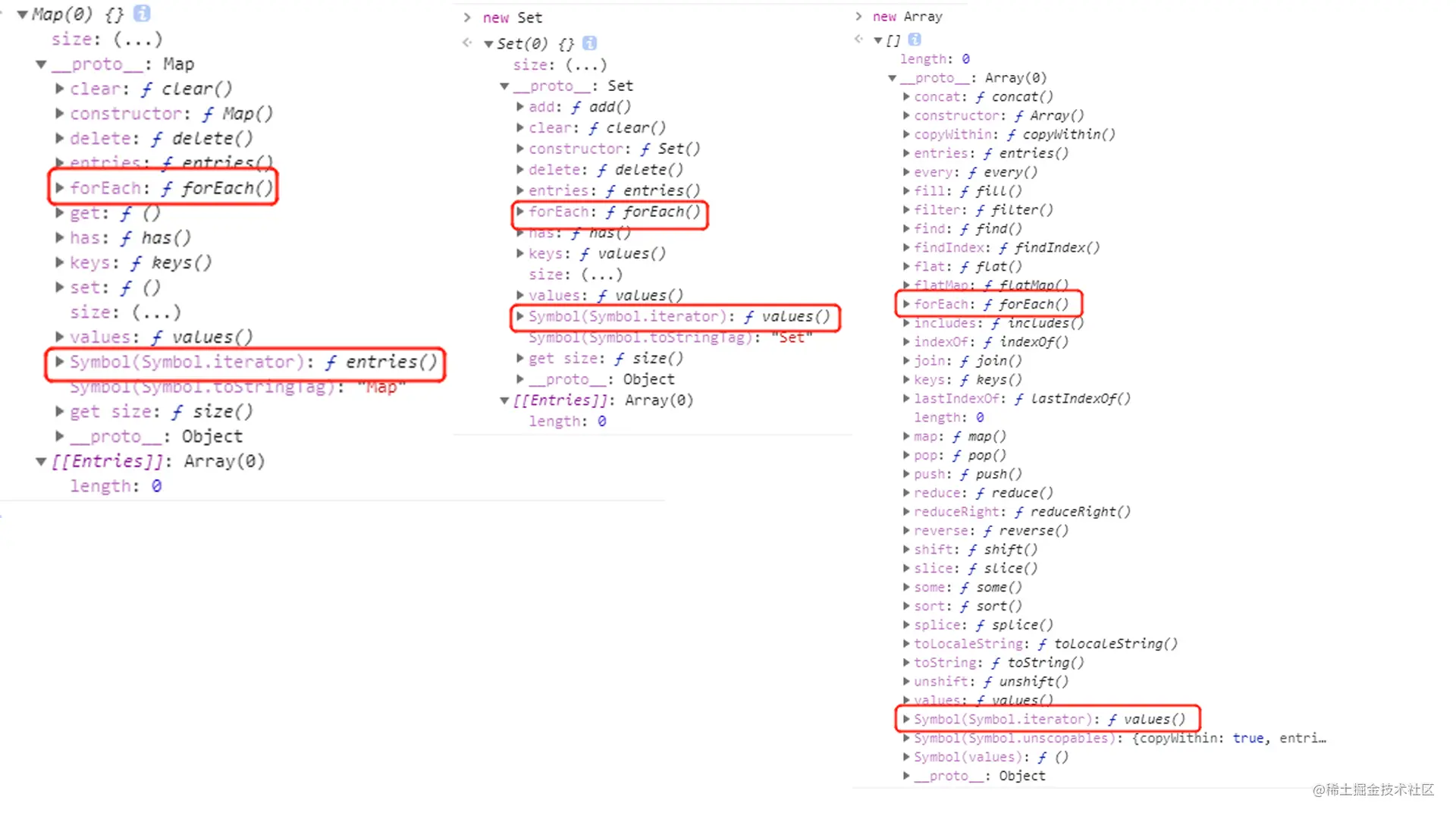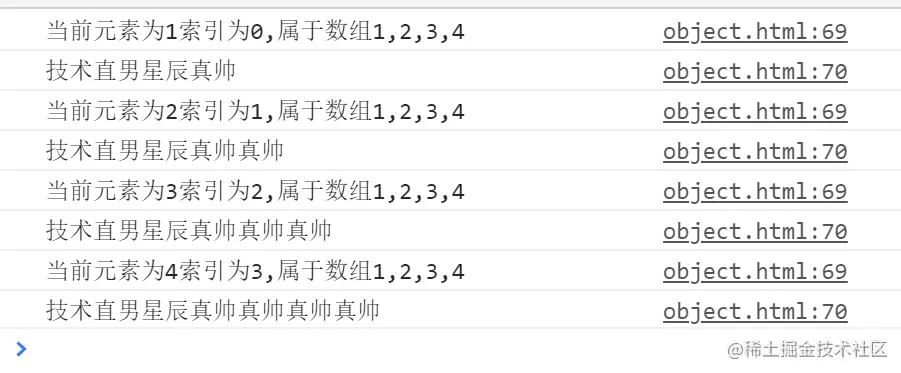# 有了for循环 为什么还要forEach？

js中那么多循环，`for` `for...in` `for...of` `forEach`，有些循环感觉上是大同小异今天我们讨论下`for`循环和`forEach`的差异。

`for`循环和`forEach`的本质区别。

`for`循环和`forEach`的语法区别。

`for`循环和`forEach`的性能区别。

### 本质区别

`for`循环是js提出时就有的循环方法。`forEach`是ES5提出的，挂载在可迭代对象原型上的方法，例如`Array` `Set` `Map`

`forEach`是一个迭代器，负责遍历可迭代对象。那么遍历迭代可迭代对象分别是什么呢。``````let arr = [1, 2, 3, 4]  // 可迭代对象
let iterator = arr[Symbol.iterator]()  // 调用 Symbol.iterator 后生成了迭代器对象
console.log(iterator.next()); // {value: 1, done: false}  访问迭代器对象的next方法
console.log(iterator.next()); // {value: 2, done: false}
console.log(iterator.next()); // {value: 3, done: false}
console.log(iterator.next()); // {value: 4, done: false}
console.log(iterator.next()); // {value: undefined, done: true}``````

``````let arr = [1, 2, 3, 4]
for (const item of arr) {
console.log(item); // 1 2 3 4
}``````

``````function num(params) {
console.log(arguments); // Arguments(6) [1, 2, 3, 4, callee: ƒ, Symbol(Symbol.iterator): ƒ]
let iterator = arguments[Symbol.iterator]()
console.log(iterator.next()); // {value: 1, done: false}
console.log(iterator.next()); // {value: 2, done: false}
console.log(iterator.next()); // {value: 3, done: false}
console.log(iterator.next()); // {value: 4, done: false}
console.log(iterator.next()); // {value: undefined, done: true}
}
num(1, 2, 3, 4)
let set = new Set('1234')
set.forEach(item => {
console.log(item); // 1 2 3 4
})
let iterator = set[Symbol.iterator]()
console.log(iterator.next()); // {value: 1, done: false}
console.log(iterator.next()); // {value: 2, done: false}
console.log(iterator.next()); // {value: 3, done: false}
console.log(iterator.next()); // {value: 4, done: false}
console.log(iterator.next()); // {value: undefined, done: true}``````

（感兴趣的同学可以搜下 `forEach` 源码， `Array` `Set` `Map` 实例上都挂载着 `forEach` ，但网上的答案大多数是通过 `length` 判断长度， 利用`for`循环机制实现的。但在 `Set` `Map` 上使用会报错，所以我认为是调用的迭代器，不断调用 `next`，传参到回调函数。由于网上没查到答案也不妄下断言了，有答案的人可以评论区给我留言）

### `for`循环和`forEach`的语法区别

1. `forEach` 的参数。
2. `forEach` 的中断。
3. `forEach` 删除自身元素，index不可被重置。
4. `for` 循环可以控制循环起点。

#### `forEach` 的参数

``arr.forEach((self,index,arr) =>{},this)``

self： 数组当前遍历的元素，默认从左往右依次获取数组元素。

index： 数组当前元素的索引，第一个元素索引为0，依次类推。

arr： 当前遍历的数组。

this： 回调函数中this指向。

``````let arr = [1, 2, 3, 4];
let person = {
name: '技术直男星辰'
};
arr.forEach(function (self, index, arr) {
console.log(`当前元素为\${self}索引为\${index},属于数组\${arr}`);
console.log(this.name+='真帅');
}, person)``````

``````let arr1 = [1, 2, 1, 3, 1];
let arr2 = [];
arr1.forEach(function (self, index, arr) {
arr.indexOf(self) === index ? arr2.push(self) : null;
});
console.log(arr2);   // [1,2,3]``````#### `forEach` 的中断

``````let arr = [1, 2, 3, 4],
i = 0,
length = arr.length;
for (; i < length; i++) {
console.log(arr[i]); //1,2
if (arr[i] === 2) {
break;
};
};
arr.forEach((self,index) => {
console.log(self);
if (self === 2) {
break; //报错
};
});
arr.forEach((self,index) => {
console.log(self);
if (self === 2) {
continue; //报错
};
});``````

``````try {
var arr = [1, 2, 3, 4];
arr.forEach(function (item, index) {
//跳出条件
if (item === 3) {
throw new Error("LoopTerminates");
}
//do something
console.log(item);
});
} catch (e) {
if (e.message !== "LoopTerminates") throw e;
};``````

``````let arr = [1, 2, 3, 4];
function find(array, num) {
array.forEach((self, index) => {
if (self === num) {
return index;
};
});
};
let index = find(arr, 2);// undefined``````

#### `forEach` 删除自身元素，index不可被重置

`forEach` 中我们无法控制 `index` 的值，它只会无脑的自增直至大于数组的 `length` 跳出循环。所以也无法删除自身进行`index`重置，先看一个简单例子：

``````let arr = [1,2,3,4]
arr.forEach((item, index) => {
console.log(item); // 1 2 3 4
index++;
});``````

`index`不会随着函数体内部对它的增减而发生变化。在实际开发中，遍历数组同时删除某项的操作十分常见，在使用`forEach`删除时要注意。

#### `for` 循环可以控制循环起点

``````let arr = [1, 2, 3, 4],
i = 1,
length = arr.length;
for (; i < length; i++) {
console.log(arr[i]) // 2 3 4
};``````

``````let arr = [1, 2, 1],
i = 0,
length = arr.length;
for (; i < length; i++) {
// 删除数组中所有的1
if (arr[i] === 1) {
arr.splice(i, 1);
//重置i，否则i会跳一位
i--;
};
};
console.log(arr); // 
//等价于
var arr1 = arr.filter(index => index !== 1);
console.log(arr1) // ``````

### `for`循环和`forEach`的性能区别

`for`：for循环没有额外的函数调用栈和上下文，所以它的实现最为简单。

`forEach`：对于forEach来说，它的函数签名中包含了参数和上下文，所以性能会低于 `for` 循环。

`map``map` 最慢的原因是因为 `map` 会返回一个新的数组，数组的创建和赋值会导致分配内存空间，因此会带来较大的性能开销。如果将`map`嵌套在一个循环中，便会带来更多不必要的内存消耗。

``````let data = [];
let data2 = [1,2,3];
data2.map(item=>data.push(item));``````